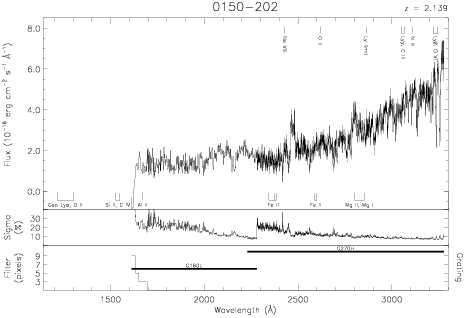Download dataset
TELESCOP= 'HST     '
INSTRUME= 'FOS     '
EQUINOX =               2000.0
DATE-OBS= '1990-10-28T10:22:46'
RA_PNT  =        28.1136817143
DEC_PNT =       -20.0189036890
PA_PNT  =       130.9192891184
OBJECT  = '0150-202'
ROOTNM01= 'Y0DK0104R'
GRNDMD01= 'SPECTROSCOPY'
DETECT01= 'AMBER   '
APERID01= 'B-3     '
FGWAID01= 'L15     '
ROOTNM02= 'Y0DK0105R'
GRNDMD02= 'SPECTROSCOPY'
DETECT02= 'AMBER   '
APERID02= 'B-3     '
FGWAID02= 'L15     '
ROOTNM03= 'Y0DK0106T'
GRNDMD03= 'SPECTROSCOPY'
DETECT03= 'AMBER   '
APERID03= 'B-3     '
FGWAID03= 'L15     '
ROOTNM04= 'Y0UR0302T'
GRNDMD04= 'SPECTROSCOPY'
DETECT04= 'AMBER   '
APERID04= 'A-1     '
FGWAID04= 'H27     '
ROOTNM05= 'Y0UR0303T'
GRNDMD05= 'SPECTROSCOPY'
DETECT05= 'AMBER   '
APERID05= 'A-1     '
FGWAID05= 'H27     '